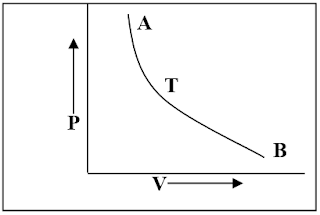# Explain isothermal and adiabatic process?### Isothermal process:

If a system is perfectly conducting to the surroundings and the temperature remains constant throughout the process, then the process is called an isothermal process. In this process, although the temperature remains constant but the heat is variable.From the given figure, from A to B, pressure decreased and work is done. There should be fall in temperature but the system absorbs heat and maintains a constant temperature.

From B to A, pressure increased and external work is done. There should be rise in temperature, but the system gives up extra heat to the surroundings and the temperature remain constant.

The equation for an isothermal process is, PV = RT = constant

In adiabatic process the working substance is perfectly insulated from the surroundings. All along the process there is change in temperature.

When work is done on the working substance, there is rise in temperature, because, the external work done on the working substance increases the internal energy. When work is done by the working substance, it is done on the cost of its internal energy, that’s why, there is fall in temperature.

The equation for an adiabatic process is, PVy  = constant